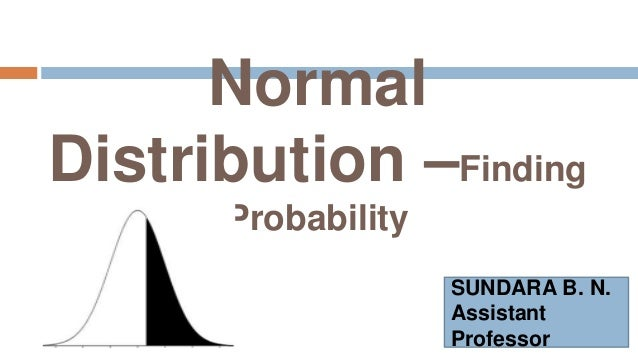Successfully reported this slideshow.
We use your LinkedIn profile and activity data to personalize ads and to show you more relevant ads. You can change your ad preferences anytime.Upcoming SlideShare
Loading in …5
×
• Full Name
Comment goes here.

Are you sure you want to Yes No
Your message goes here• Be the first to comment

• Be the first to like this

### Normal Distribution - Find the Probability

1. 1. Normal Distribution –Finding Probability SUNDARA B. N. Assistant Professor
2. 2. Problem ABC ltd., is considering a project. The projected average cash out flow is Rs. 600 Lakh with a Standard Deviation Rs. 40 Lakh. Calculate a) The probability of cash flow being less than Rs. 660 Lakh b) The probability of cash flow being more than Rs. 500 Lakh c) The probability of cash flow being between Rs. 480 lakh and Rs. 560 lakh
3. 3. Given Information Here we are given that μ = Rs. 600 Lakh σ = Rs. 40 Lakh  X~N(600, 1600), We know that is X~N(μ, σ²), then the S.N.V is given by Z = X – μ σ
4. 4. i) The probability of cash flow being less than Rs. 660 Lakh Consider X = 660  P(X<660)=P(Z<1.5 ) = 0.5+P(0<Z<1.5) = 0.5+0.4332(TV) =0.9332 The probability of cash flow being less than Rs. 660 Lakh = 0.9332 Z=1.5 Z=0 X=600 X=660
5. 5. ii) The probability of cash flow being more than Rs. 500 Lakh Consider X = 500  P(X>500)=P(Z>-2.5) =P(Z>2.5) = 0.5+P(0<Z<2.5) = 0.5+0.4938 (TV) =0.9938 The probability of cash flow being more than Rs. 500 Lakh =0.9938 Z=0 Z=-2.5 X=600 X=500
6. 6. iii) The probability of cash flow being between Rs. 480 lakh and Rs. 560 lakh Consider X = 480 and X = 560  P(480<X<560)=P(-3<X<- 1) = P(-3<X<0)-P(0<Z<-1) = P(0<Z<3)-P(0<Z<1) = 0.4987-0.3413 (TV) =0.1574 The probability of cash flow being between Rs. 480 lakh and Rs. 560 lakh =0.1574 Z=0 Z=-1 Z=-3 X=480 X=600 X=560